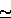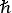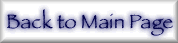## 2. Form Factors and Standard Definitions

The real part of the atomic x-ray scattering factor is(eq 3)

where f′(E,Z) =f+ + frel is composed of the non-relativistic anomalous dispersion f+ and the relativistic correction factor frel.     f0(q,Z) is the `normal' coherent scattering factor as a function of momentum transfer q = |Kf - Ki| = (4πsin θ/λ), the latter relation for elastic scattering through an angle 2θ.(eq 4)

The dependences of f upon q and E may not be strictly separable as implied by the separation into f0 and f′. Because of this, some authors define a modified form factor MFF (g)  or anomalous scattering factors (g′ and g″) . The nuclear Thomson scattering (NT) is small and negative in phase relative to the electronic form factor (f0+f′), of magnitude fNT = -Z2m/M, with small (negligible) energy and momentum dependence [13,14]. The S-matrix channels of nuclear photo-effect (dipole resonance), pair-N, pair-e production and Delbrück scattering and other, smaller effects are typically neglected, providing upper limits in energy for the validity of these syntheses.

The imaginary part of the dispersion correction is simply linked to the photoeffect cross section µPE(E) (neglecting, or including, bound-bound cross-sections) at the photon energy E:(eq 5)

f1 and f2 are used in Refs. [7,15,16]. The form factor f1 is particularly useful in illustrating effects described herein because it approximates Re(f) in the forward scattering direction where f0Z and hence estimates the total scattering factor, placing the problems indicated on an absolute footing. It is also appropriate in typical experiments. The standard dispersion relation yields(eq 6)

Here E =ω is the x-ray energy, ε′ is the energy above the electron binding energy of the intermediate (bound or continuum) state, and P represents the principal value. Commonly the equation may be separated by orbital or by electron, with neglect of unoccupied bound states yielding(eq 7)

where -ε1 is the electron binding energy, and ε+ is the (continuum) energy. The high-energy constant may be combined with relativistic correction factors to provide the separation into frel and f+ as indicated above.